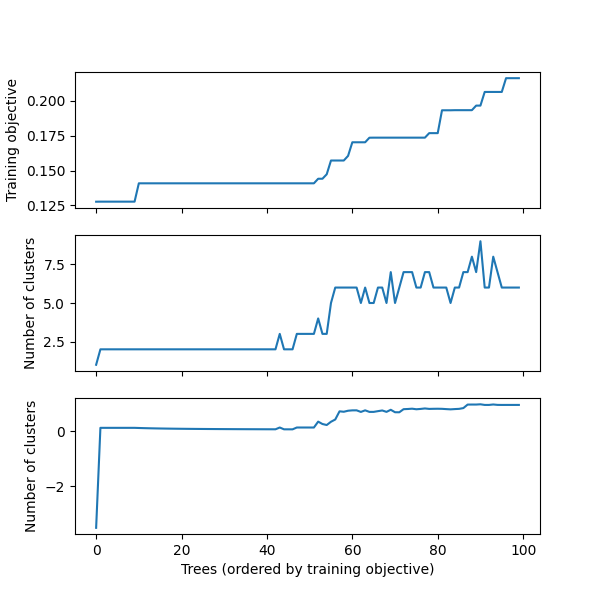# Differences between Python and Julia

The IAI Python interface matches the Julia API very closely, so you can refer to the Julia documentation for information on most tasks. On this page we note the main differences between the Python and Julia interfaces.

## Conversion of Julia data types to Python

In order to figure out the types to pass to an IAI function from the Python interface, you can refer to the equivalent function in the Julia API and translate the types to their Python equivalent. Most literal data types convert in a straighforward manner, for example:

• Int to int
• Float64 to float
• String to str
• Dict to dict

The following Julia types can be passed as follows:

• nothing can be passed using None
• a Symbol can be passed as a str
• a Vector can be passed as a list, 1-D numpy.array or a pandas.Series
• a Matrix can be passed as a 2-D numpy.array
• a DataFrame can be passed as a pandas.DataFrame

### Specifying Feature Set in Python

We list the Python input types for specifying set of features in a dataframe as learner parameters. Refer to IAI.FeatureSet for the Julia equivalence. Note that if you are using integers to specify the indices of columns, the input is expected to use one-based indexing as in Julia.

Input TypeDescriptionExamples
AllUse all columns{'All'}, {'All' : []}
Integer or a vector of IntegersSpecify indices of columns to use1, [1, 3, 4]
String or a vector of StringsSpecify names of columns to use'x1', ['x1', 'x3']
NotSpecify columns not to use{'Not' : 1}, {'Not' : ['x2', 'x4']}
BetweenSpecify range of columns to use{'Between' : ['x1', 'x4']}

## Object-oriented interface for learners

In the IAI Python interface, the API methods relating to learners are methods of the learner objects rather than functions that operate on learners as in the Julia interface. For instance the IAI.fit! method in Julia:

IAI.fit!(lnr, X, y)

would be called from the Python interface as

lnr.fit(X, y)

## Interactive Visualizations

The write_html and show_in_browser functions work the same in Python as in Julia for saving visualizations to file or displaying in an external browser, respectively. Additionally, visualizations will be automatically shown in Jupyter notebooks as they are for Julia.

Below is an example that shows the equivalent Python code for the advanced visualization examples in Julia. In these examples we work with the following tree learner:

Optimal Trees Visualization

We can rename the features with a dict that maps from the original names to more descriptive names:

vis_renamed_features = lnr.TreePlot(feature_renames={
"Disp": "Displacement",
"HP": "Horsepower",
"WT": "Weight",
})

Optimal Trees Visualization

We can also have a finer-grained control of what is displayed for each node, such as adding summary statistics. We create a list of dicts with the parameters controlling what you want to show in each node and pass this as extra_content:

import numpy as np
node_inds = lnr.apply_nodes(X)
def get_text(inds):
content = ('<b>Mean horsepower in node:</b> ' +
str(np.round(np.mean(X.HP.iloc[inds]), decimals=2)))
return ({'node_details_extra' : content})
extras = [get_text(inds) for inds in node_inds]
vis_extra_text = lnr.TreePlot(extra_content=extras)

Optimal Trees Visualization

Finally, we can combine multiple learners in a single visualization as described in the Julia documentation. In Python, a question is a tuple of the form (question, responses), where question is the string prompt for the question and responses is a list of possible responses:

questions = ("Use learner with", [
("renamed features", vis_renamed_features),
("extra text output", vis_extra_text)
])
iai.MultiTreePlot(questions)

Optimal Trees Visualization

### Tree Stability

Below are examples showing the equivalent Python code for the tree stability examples in Julia. In these examples we work with the following tree learner:

Optimal Trees Visualization

#### Stability Analysis

We conduct the stability analysis using StabilityAnalysis:

stability = iai.StabilityAnalysis(lnr, train_X, train_y, criterion='gini')


We can plot a summary of the analysis using plot:

stability.plot()We can use get_stability_results to extract the trees in order of training objective along with the importance of each feature in the tree:

stability.get_stability_results()

    train_error  tree_index  variance  skewness  curtosis  entropy
0      0.127639          38  0.601713  0.395491  0.002795      0.0
1      0.127677          21  0.615924  0.237532  0.146544      0.0
2      0.127677          22  0.615924  0.237532  0.146544      0.0
3      0.127677          29  0.615924  0.237532  0.146544      0.0
4      0.127677          39  0.615924  0.237532  0.146544      0.0
5      0.127677          55  0.615924  0.237532  0.146544      0.0
6      0.127677          59  0.615924  0.237532  0.146544      0.0
..          ...         ...       ...       ...       ...      ...
93     0.206366          36  0.783420  0.185882  0.030698      0.0
94     0.206366          57  0.783420  0.185882  0.030698      0.0
95     0.206366          76  0.783420  0.185882  0.030698      0.0
96     0.216240          87  0.746042  0.195427  0.058531      0.0
97     0.216240          25  0.764514  0.196132  0.039354      0.0
98     0.216240          30  0.764514  0.196132  0.039354      0.0
99     0.216240         100  0.764514  0.196132  0.039354      0.0

[100 rows x 6 columns]

We can use get_cluster_details to summarize the clustering of the first 10 trees:

stability.get_cluster_details(10)

   train_error_mean  variance  skewness  curtosis  entropy
0          0.127639  0.601713  0.395491  0.002795      0.0
1          0.127677  0.615924  0.237532  0.146544      0.0

We can use get_cluster_distances to get the relative distances between each pair of clusters:

stability.get_cluster_distances(10)

array([[0.        , 0.21404831],
[0.21404831, 0.        ]])

We can use get_cluster_assignments to see which trees comprise each cluster:

stability.get_cluster_assignments(10)

[array(, dtype=int64), array([21, 22, 29, 39, 55, 59, 70, 96, 97], dtype=int64)]

We see that Tree 38 is in its own cluster, and the other trees are all grouped together. Given this, we might want to inspect how Tree 38 differs to the others. We can use get_tree to construct a new learner that uses the tree at a specified index:

lnr.get_tree(38)

Optimal Trees Visualization